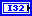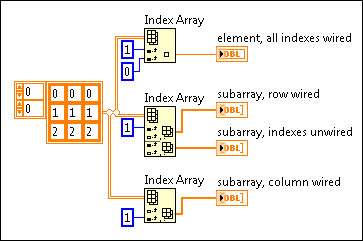Index Array Function

FlexRIO Help

Edition Date: November 2015

Part Number: 372614J-01

»View Product Info

Owning Palette: Array Functions

Requires: Base Development System

Returns the element or subarray of n-dimension array at index.

When you wire an array to this function, the function resizes automatically to display index inputs for each dimension in the array you wire to n-dimension array. You also can add additional element or subarray terminals by resizing the function. The connector pane displays the default data types for this polymorphic function.n-dimension array can be an n-dimensional array of any type. If n-dimension array is an empty array, element or subarray returns the default value of the defined data type for the array.index 0..n-1 specifies a number that refers to a location within the input array. LabVIEW automatically provides an index input for each dimension of the array.

Behavior for Out of Range Values

If the index is less than zero or greater than the number of dimensions in array, this function returns the default value of the defined data type for the array.

Which Index Refers to Which Dimension?

Array functions in LabVIEW access array data in row-major order. In a 2D array, row is the first, major index. Column is the last, minor index. In bigger multidimensional arrays, column remains the last index, and this function adds the more major indexes to the front. Thus, vol is the first index, followed by page, row, and column. These names are index identifiers and have no other meaning.

Unwired Index Inputs

Unwired index inputs allow you to retrieve a subarray of the array rather than a single element. For example, to retrieve column 1 of a 2D array, specify 1 in the column index and leave the row index unwired.

If you leave the index input unwired for a 1D array, the Index Array function returns the first element of the array.

How Does the Index Relate to Multiple Outputs?

If you expand the node to show more than one element or subarray output, LabVIEW provides a set of index inputs for each output. The values you wire to a set of index inputs determine the value of the corresponding output. However, if you do not wire values to a set of index inputs, the corresponding element or subarray output returns the element or subarray that follows the previous element or subarray output in the original array. Refer to the Examples section for an illustration of this relationship.element or subarray has the same type as the elements of n-dimension array.

Index Array Details

You can disable indexing along a dimension by leaving the corresponding index input unwired, unless you are indexing a 1D array. By default, the first dimension has indexing enabled, with the others disabled. If disabled, the input terminal is an outlined black rectangle. If enabled, it is filled. You can wire a constant or control to the index inputs that you want enabled.

For example, if you want to index a row in a 2D array, the first index input is enabled and the second index input is disabled. If you want to index that same 2D array also by column, you can resize the function to show another set of input terminals. This next set of inputs has its own corresponding subarray output. By default, if you do not wire any index input terminals, the first subarray indexes row 0, the second subarray indexes row 1, and so on.

Examples of Different Input Configurations

The following image and table illustrate this function's behavior for a variety of input values: